Reach Us+32-10-28-02-25
Inertia effect on free convection over horizontal surface in a porous medium with a variable wall heat flux and variable wall temperature containing Internal heat generation
Journal of Physical Mathematics
All submissions of the EM system will be redirected to Online Manuscript Submission System. Authors are requested to submit articles directly to Online Manuscript Submission System of respective journal.

# Inertia effect on free convection over horizontal surface in a porous medium with a variable wall heat flux and variable wall temperature containing Internal heat generation

Ferdows M1* and Liu D2

1Department of Applied Mathematics, University of Dhaka, Bangladesh

2Department of Engineering and Science, Louisiana Tech University, USA

*Corresponding Author:
Ferdows M
Professor, Department of Mathematics
Tel: 88-9672533
E-mail:
[email protected]

Received date: February 03, 2016; Accepted date: April 28, 2016; Published date:May 02, 2016

Citation: Ferdows M, Liu D (2016) Inertia effect on free convection over horizontal surface in a porous medium with a variable wall heat flux and variable wall temperature containing Internal heat generation. J Phys Math 7: 165. doi:10.4172/2090-0902.1000165

Copyright: © 2016 Ferdows M, et al. This is an open-access article distributed under the terms of the Creative Commons Attribution License, which permits unrestricted use, distribution, and reproduction in any medium, provided the original author and source are credited.

Visit for more related articles at Journal of Physical Mathematics

#### Abstract

The analysis is performed for the steady-state boundary layer flow with free convective heat transfer over a horizontal surface embedded in a fluid-saturated porous medium subject to variable wall heat flux (WHF) and variable wall temperature (VWT). In modeling the flow inertia, no slip boundary conditions and exponentially decaying internal heat generation (IHG) are taken into account. The similarity equations are solved numerically and their solutions are dependent on the problem parameters G, m, λ. The influence of such parameters on flow characteristics such as velocity, temperature profiles, the skin friction coefficient, and the Nusselt number are thoroughly discussed. It is found that the flow profiles along with physical parameters significantly altered the rate of heat transfer and induced more flow within the boundary layer than that of without IHG.

#### Keywords

Inertia; Nusselt number; Rayleigh number

#### Introduction

Numerous phenomena involve free convection driven by internal heat generation. Free convection, as a process of vital significance, has been of interest to researchers in both industry and academia, such as a recent numerical work by Liu et al. . Two important modelling of free convection are related to nuclear energy and combustion, specifically in the development metal waste from spent nuclear fuel and the storage of spent nuclear fuel, see Horvat et al. , Jahn and Reinke . A couple of recent papers have been devoted to the subject of similarity solutions for free with internal heat generation in porous media for several geometric configurations, see Bagai , Postelnicu and Pop , Postelnicu et al. [6,7]. Several recent papers, such as Magyari et al. [8,9], Mealey and Merkin , Merkin  throw new lights on the internal heat generation in porous media, remaining in the frame of similar solutions.

Turning now to the non-Darcy formulations, with focus on vertical and horizontal configurations in porous media, we present below a short review of the literature dedicated on these topics.

a) Vertical configuration

• Thermal dispersion, which becomes prevalent in the non- Darcy regime, and viscous dissipation effects are studied by Murthy ) for a vertical flat plate configuration, with inertia effect included in the momentum equation. Constant wall temperature conditions were considered in that study.

• Nonsimilarity solutions for the non-Darcy mixed convection from a vertical flat plate embedded in a saturated porous medium for a power-law variation of the wall temperature were reported by Kodah and Duwairi .

• A number of non-Darcian effects, including the high-flowrate inertia were analysed by Hung and Chen  for a free convection along a impermeable vertical plate embedded in a thermally stratified fluid-saturated porous medium. Variable heat flux conditions are imposed in that study.

b) Horizontal configurations

• The presence of inertia (Forchheimer form-drag) in the steady free convection boundary layer over an upward-facing horizontal embedded in a porous medium was studied by Rees .

• This work was extended later by Hossain and Rees  by taking the surface temperature as a power-law variation in terms of the distance from the leading edge.

• Duwairi et al.  analyzed the combined free and forced convection regimes within a non-Darcy model for a horizontal configuration.

In view of the above literature survey, it seems that the effect of inertia on free convection from a horizontal surface embedded in a porous medium with internal heat generation was not considered till now, at our best knowledge. Two cases are considered in the paper: variable wall heat flux and variable wall temperature. Distinctive from the previous quoted studies where inertia effects have been considered, in the present paper there are obtained similarity solutions, in the spirit of studies dealing with internal heat generation.

#### Analysis

Consider the natural convection , laminar, two dimensional boundary layer flow over horizontal surfaces in porous media with internal heat generation of wall temperature Tw and uniform ambient temperature T∞,where Tw > T∞. The x-coordinate is measured along the surface and the y-coordinate normal to it. Under the Boussinesq and boundary layer approximations, the basic equations are continuity equation, momentum equation and energy equation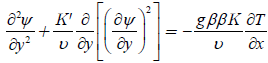(1)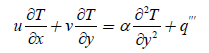(2)

where the stream function Ψ was introduced through the relationships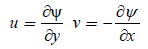(3)

such as the continuity equation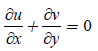(4)

Is identically satisfied.

Case of variable wall heat flux

The boundary conditions for the model are

υ=0, qw(x)=axm, at y = 0 (5a)

u=0, T=T, as y→ (5b)

We now introduce the following dimensionless variables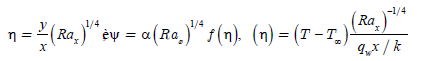(6)

Where the local Rayleigh number is defined as(7)

We then consider the internal heat generation (IHG) of the form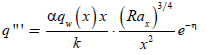(8)

Equations (1) and (2) become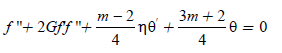(9)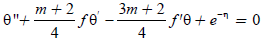(10)

where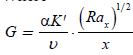(11)

Is the inertia parameter. The corresponding boundary conditions are

f(0)=0, θ(0)=-1 (12a)

f(∞)=0, θ(∞)=0 (12b)

Quantities of engineering interest are the skin friction coefficient and Nusselt number, defined as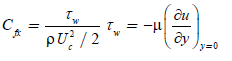(13)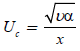(14)

where Uc is a characteristic velocity, taken here as(15)

Performing the calculations, we get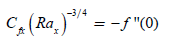(16)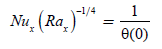(17)

Case of variable wall temperature

1. The boundary conditions in this case are

υ=0, T=Tw(x)=T+Axλ, at y = 0(18a)

u=0, T=T, as y→∞ (18b)

We now introduce the following dimensionless variables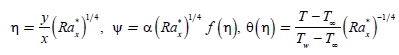(19)

Where the local Rayleigh number is defined as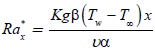(20)

Now, the internal heat generation term is taken in the following form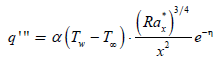(21)

in order to obtain similarity solutions.

The problem becomes, in transformed variables,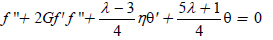(22)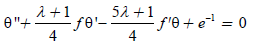(23)

That must be solved along the boundary conditions

f(0)=0, θ(0)=1 (24a)

f'(∞)=0, θ(∞)=0 (24b)

The expression of the skin friction coefficient remains the same, so that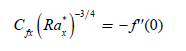(25)

While for the Nusselt number we get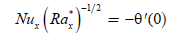(26)

Numerical Analysis and Results

The two sets of boundary value problems (9), (10), (12) and (22-24) were solved by two methods.

a) A well-known finite difference method, namely the Kellerbox method, incorporated in our own code

b) Using the dsolve routine from MAPLE .

The results given by these methods were compared and in each case the maximum value of η, say ηmax, was adjusted in order to conciliate the outputs and get grid independence in case a). As a rule, the dynamic and thermal boundary layers become thicker as G increases and also the convergence of the numerical procedure is achieved with more difficulty. Basically, ηmax was raised till 20, when G was increased. Results are reported separately for the two cases considered in this study: Figures 1-6 are variable surface heat flux (VHF), while Figures 7-12 for variable wall temperature boundary conditions (VWT). The variations of the skin friction and Nusselt number with the inertia parameter are shown in Figures 1 and 2 respectively considering with and without IHG. From Figure 1, it is seen that in both the cases (with and without IHG) the skin friction is more significant and induced more flow than that of without IHG, hence give rise to the velocity gradient leads to the increase of the skin friction. On the other hand, one can easily remark from Figure 2 that the Nusselt number with IHG is less intensive than the presence of IHG.

Figure 3:Velocity profiles in VHF case, without internal heat generation, for several values of G and m.

Figure 4: Velocity profiles in VHF case, internal heat generation, for several values of G and m.

Figure 5: Temperature profiles in VHF case, without internal heat generation, for several values of G and m.

Figure 6: Temperature profiles in VHF case, internal heat generation, for several values of G and m.

Figures 3-6 presents the solutions of the dimensionless velocity and temperature profiles for several values of G and m considering with and without IHG. We can see that the velocity profiles decreases with increasing G (and m). The reverse happens as one move far away from the surface indicating that the effects of the parameters are more dominant near the surface. Further it is also seen that the momentum boundary layer thickness has influenced greatly by IHG. From Figures 5 and 6, it is seen that the temperature profiles increases and tends to zero at the edge of the thermal boundary layer satisfying the boundary condition θ()=0. Also the thermal boundary layer thickness increases when the effect of IHG is considered. The dimensionless velocity and temperature profiles as well as ski friction coefficient and Nusselt number for some values of G and λ in case of VWT are presented in Figures 7-12. We can draw the same conclusions as in the case of VHF.5.

#### Conclusions

At our best knowledge, the available studies on free convective boundary layers in porous media with inertia effects included in non- Darcy models do not report variations of the skin friction and Nusselt number with G, due to the fact that only non-similarity solutions have been obtained. In such circumstances, the authors concentrated themselves on the variation of these two quantities along the non-similar variable (in stream wise direction). We are here in another situation, due to the existence of similarity solutions, so that we found that for both cases, VHF and VWT, a maximum value G=100 are enough to achieve an almost constant value of the skin friction and Nusselt number.

Figure 9:Velocity profiles in VWT case, without internal heat generation, for several values of G and λ.

Figure 10: Velocity profiles in VWT case, with internal heat generation, for several values of G and λ.

Figure 11: Temperature profiles in VWT case, without internal heat generation, for several values of G and λ.

Figure 12:Temperature profiles in VWT case, with internal heat generation, for several values of G and λ.

#### References

Select your language of interest to view the total content in your interested language

### Article Usage

• Total views: 9669
• [From(publication date):
June-2016 - Nov 15, 2019]
• Breakdown by view type
• HTML page views : 9454Can't read the image? click here to refresh